### Some basics of the VARIABLE block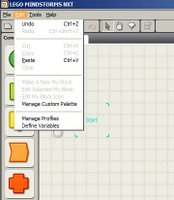From the "Edit" menu, choose Define Variables...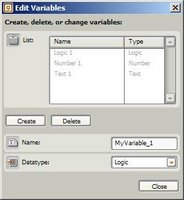I clicked the "Create" button and created a variable called Jim1.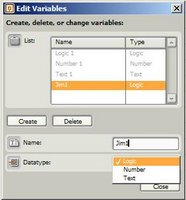My choices are Logic, Number, or Text... I choose Number.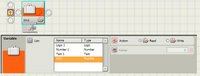I drop a VARIABLE block onto the desktop... the configuration menu allows me to select Jim1 as my variable.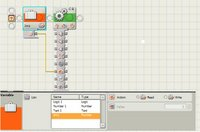Then I drop in a MOVE block... I "wire" the Jim1 variable to feed into the Power port.

Most blocks have these drop-down wiring ports... left side is input, right side is output... using these, you can use the actual values from blocks (and your own defined variables) to feed values directly into other blocks...Anonymous said…
I'm starting to envision complex programs being littered with tons of 'wires'. It might bring new meaning to 'spaghetti code'. :)Brian Davis said…
It does sometimes get very confusing, but the editor tries to sort things out automatically, and does a reasonable job. There are also ways to force the wiring into more "manual" paths if you so desire. All in all, it's a nice trade-off, but some things do end up looking like spaghetti (particularly complex math... but, hey, at least you can do it).

--
Brian DavisAnonymous said…
Assuming the ability to have 'myBlocks' have input and output wires, I would think that 'complex maths' would be a perfect candidate for such things.

How complex do the included maths get anyway? (Maybe another topic suggestion...)

+ - ?
/ * ?
DIV MOD ?
SIN() COS() TAN() ?
x^2 ?
x^y ?
SQRT(x) ?
>> << (bit shifting) ?

I'm sure there's some functions I'm forgetting, but that covers the basics of algebra, discrete mathematics, expodentials and trig.

And before you ask, yes, I've can see a use for every single one of those in various applications, most of them involving the distance sensor and the servo-rotation sensor.Anonymous said…
Here's an SQRT routine I wrote tonight in NBC. Use the SQRT macro to call it with contant or variable. Call it like SQRT(abc). Result is in sqrtResult. If you're concerned with multi-threading, use a mutex.

#define SQRT(X) mov sqrtValue, X \
subcall sqrt, sqrt_ret

// data segment
dseg segment

sqrt_ret sdword

sqrtPairs byte[] 0, 0, 0, 0, 0, 0
sqrtPaircount sbyte
sqrtValue dword
sqrtResult dword
sqrtP dword
sqrtR dword
sqrtM dword
sqrtN dword

dseg ends

/*
function: sqrt
return value: sqrtResult
params: sqrtValue
*/

// if the input value is 0, we're done
set sqrtResult, 0
brtst EQ, sqrtDone, sqrtValue

// init the paircount array
mov sqrtPaircount, 0
replace sqrtPairs, sqrtPairs, 0, 0
replace sqrtPairs, sqrtPairs, 1, 0
replace sqrtPairs, sqrtPairs, 2, 0
replace sqrtPairs, sqrtPairs, 3, 0
replace sqrtPairs, sqrtPairs, 4, 0

sqrtPairsLoop:
sqrtPairsLoop:
brtst EQ, sqrtPairsOK, sqrtValue
mod sqrtN, sqrtValue, 100
// DEBUGLOGD('PairsLoop_sqrtValue:',sqrtValue)
// DEBUGLOGD('PairsLoop_sqrtN:',sqrtN)
replace sqrtPairs, sqrtPairs, sqrtPaircount, sqrtN
div sqrtValue, sqrtValue, 100

jmp sqrtPairsLoop

sqrtPairsOK:
// get the leftmost pair
index sqrtP, sqrtPairs, sqrtPaircount
set sqrtResult, 1

// find the sqrt for the leftmost pair (1-9), if 0 we're not here!
sqrtFirstLoop:
mul sqrtN, sqrtResult, sqrtResult
brcmp GT, sqrtFirstOK, sqrtN, sqrtP
jmp sqrtFirstLoop

sqrtFirstOK:
sub sqrtResult, sqrtResult, 1
// got the sqrt of the first pair in sqrtResult

mul sqrtN, sqrtResult, sqrtResult
sub sqrtM, sqrtP, sqrtN

// in loop we get 1 new digit in sqrtResult for each pair
sqrtBigLoop:
sub sqrtPaircount, sqrtPaircount, 1

brtst LT, sqrtDone, sqrtPaircount

mul sqrtM, sqrtM, 100
index sqrtP, sqrtPairs, sqrtPaircount

// find the next digit
set sqrtN, 1

sqrtDigitLoop:
mul sqrtR, sqrtResult, 20
mul sqrtR, sqrtR, sqrtN

brcmp GT, sqrtDigitDone, sqrtR, sqrtM

jmp sqrtDigitLoop

sqrtDigitDone:
sub sqrtN, sqrtN, 1
// got the next digit

// calculate the new value to continue with
mul sqrtR, sqrtResult, 20
mul sqrtR, sqrtR, sqrtN
sub sqrtM, sqrtM, sqrtR

// add the new digit to the end of the result in sqrtResult
mul sqrtResult, sqrtResult, 10

jmp sqrtBigLoop

sqrtDone:

subret sqrt_ret
endt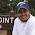JaJa Binks said…
If I have two sensor blocks of same type (say the light sensor block) connected sequentially. Does one block overwrite the intensity value of the other block if I am using the intensity output of both the block some time in the future ? Should I store the output of sensor in a variable ?